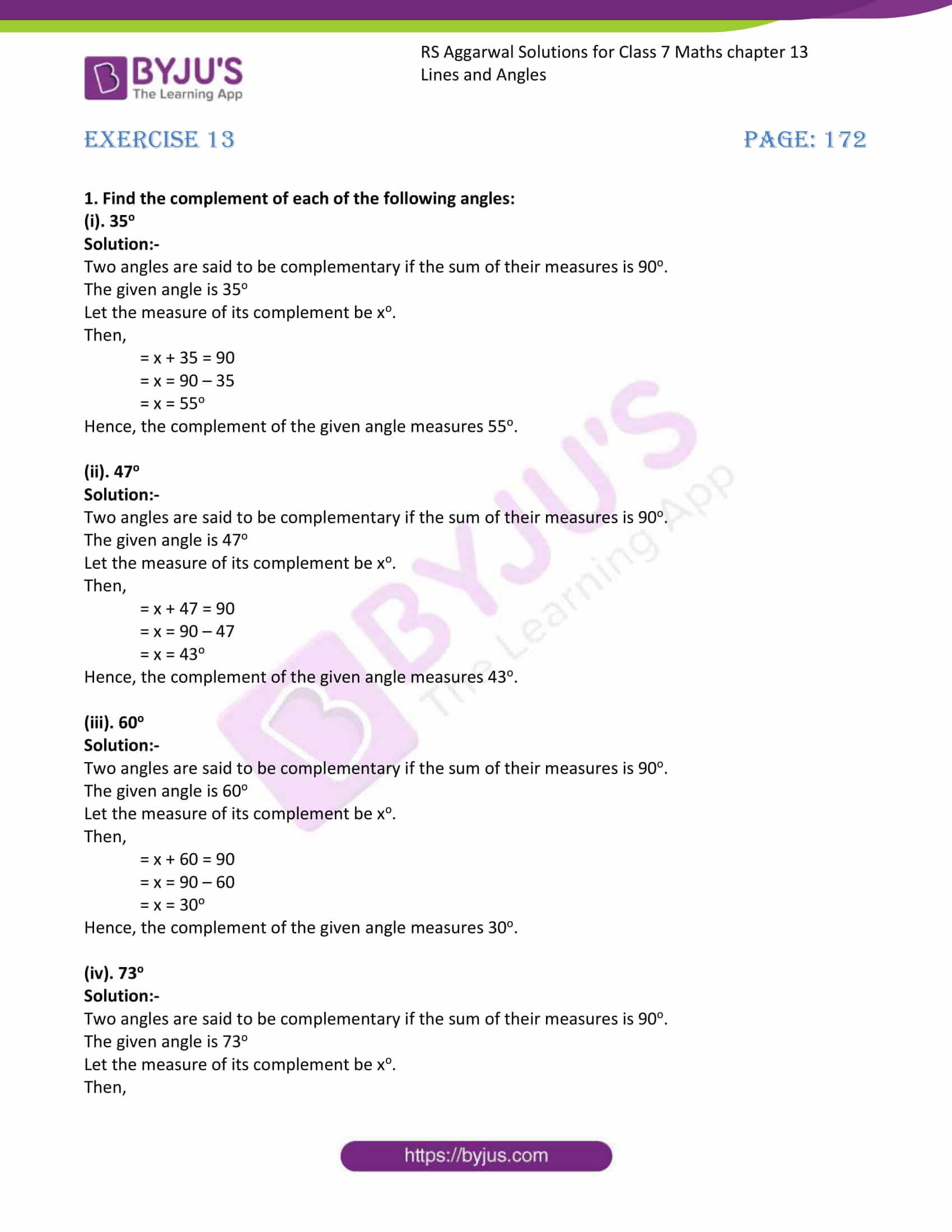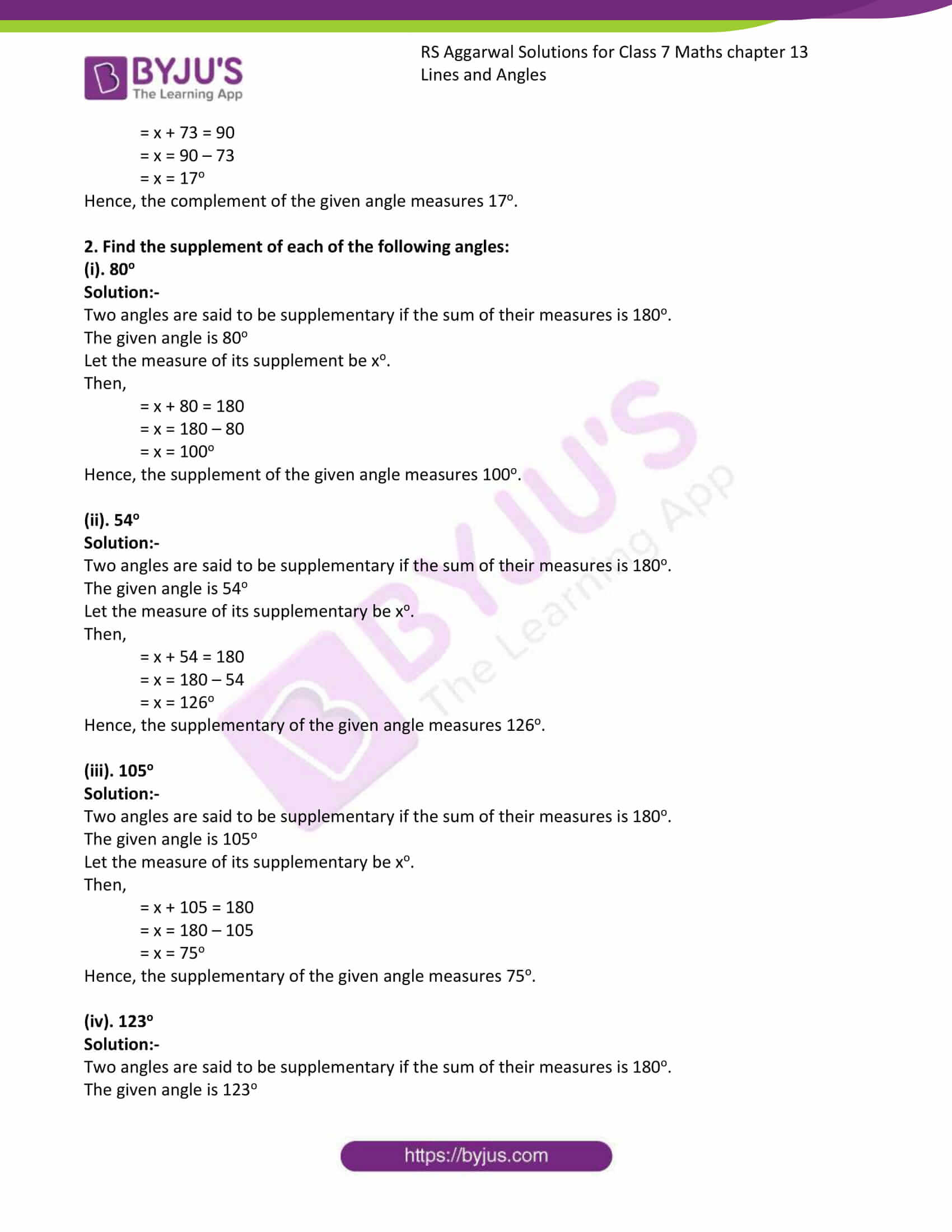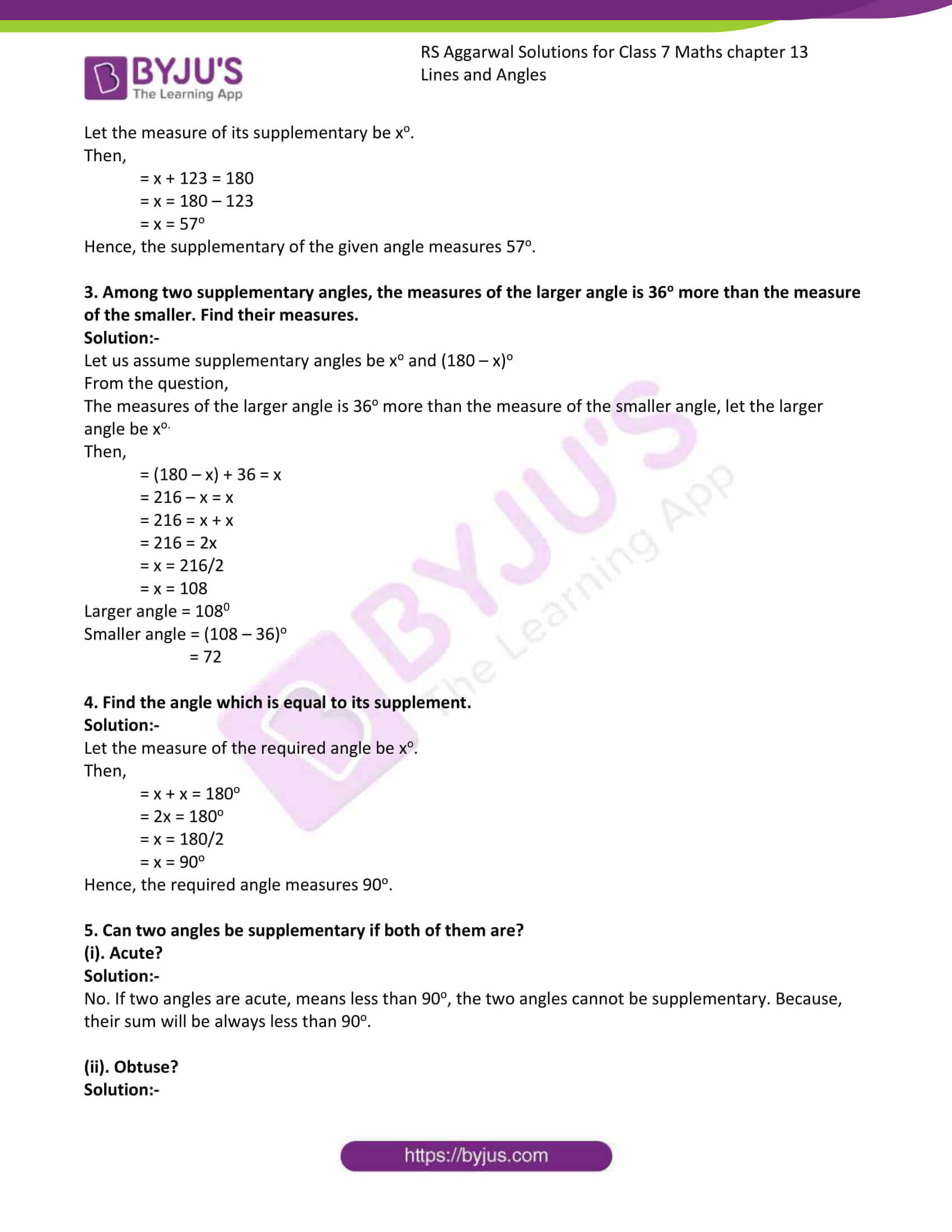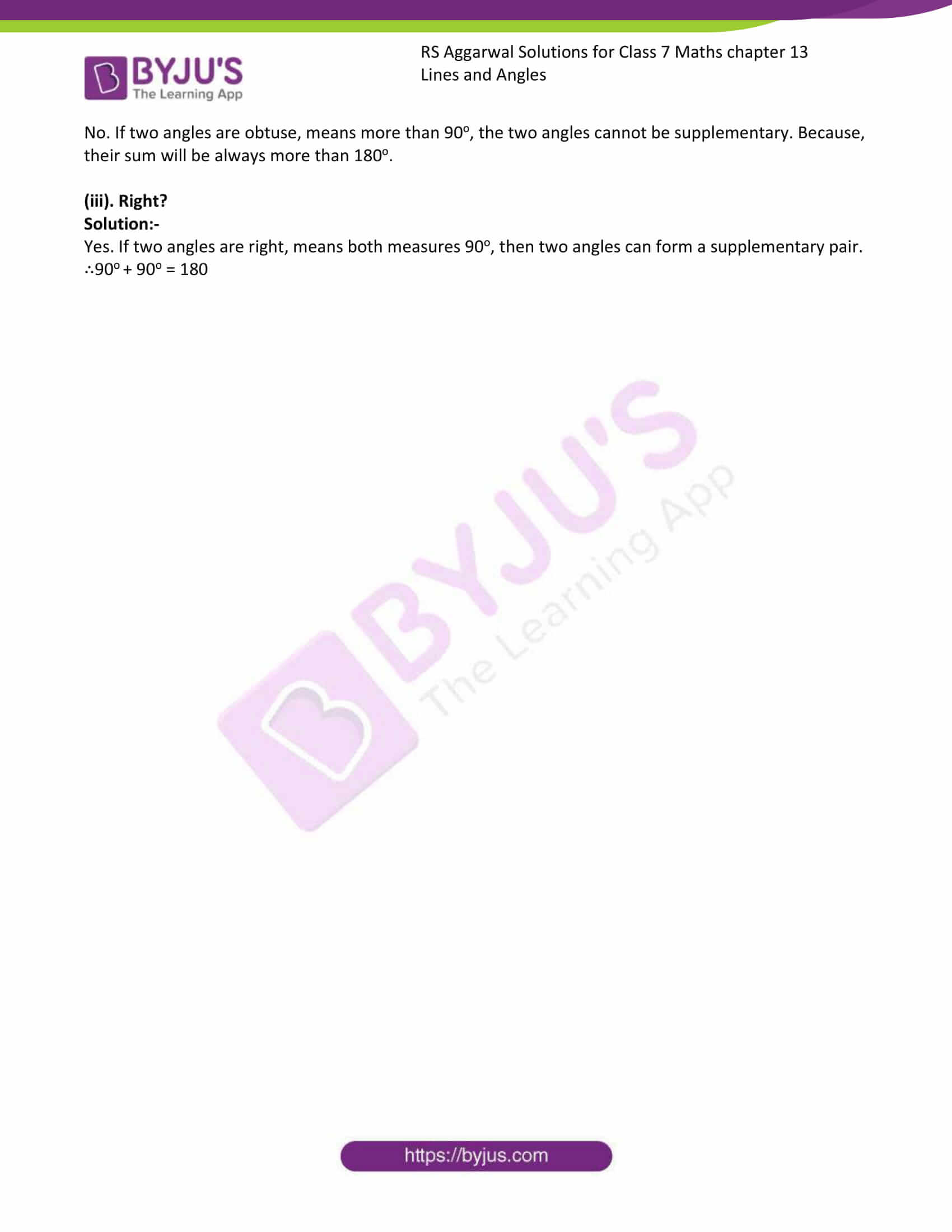# RS Aggarwal Solutions for Class 7 Maths Chapter 13 Lines and Angles

RS Aggarwal Solutions for Class 7 Maths Chapter 13 – Lines and Angles are available here. These exercises are formulated by our expert tutors in order to assist you with your exam preparation to attain good marks in Maths. Students who wish to score good marks in Maths practise RS Aggarwal Class 7 Solutions. This book is one of the top materials when it comes to providing a question bank to practice from.

The main aim is to help students understand and crack these problems. We at BYJU’S have prepared the RS Aggarwal Solutions for Class 7 Maths Chapter 13 wherein problems are solved step by step with detailed explanations. Download pdf of Class 7 Chapter 13 in their respective links.

## Download PDF of RS Aggarwal Solutions for Class 7 Maths Chapter 13 – Lines and Angles### Access answer to chapter 13 – Lines and Angles

Exercise 13

1. Find the complement of each of the following angles:

(i). 35o

Solution:-

Two angles are said to be complementary if the sum of their measures is 90o.

The given angle is 35o

Let the measure of its complement be xo.

Then,

= x + 35 = 90

= x = 90 – 35

= x = 55o

Hence, the complement of the given angle measures 55o.

(ii). 47o

Solution:-

Two angles are said to be complementary if the sum of their measures is 90o.

The given angle is 47o

Let the measure of its complement be xo.

Then,

= x + 47 = 90

= x = 90 – 47

= x = 43o

Hence, the complement of the given angle measures 43o.

(iii). 60o

Solution:-

Two angles are said to be complementary if the sum of their measures is 90o.

The given angle is 60o

Let the measure of its complement be xo.

Then,

= x + 60 = 90

= x = 90 – 60

= x = 30o

Hence, the complement of the given angle measures 30o.

(iv). 73o

Solution:-

Two angles are said to be complementary if the sum of their measures is 90o.

The given angle is 73o

Let the measure of its complement be xo.

Then,

= x + 73 = 90

= x = 90 – 73

= x = 17o

Hence, the complement of the given angle measures 17o.

2. Find the supplement of each of the following angles:

(i). 80o

Solution:-

Two angles are said to be supplementary if the sum of their measures is 180o.

The given angle is 80o

Let the measure of its supplement be xo.

Then,

= x + 80 = 180

= x = 180 – 80

= x = 100o

Hence, the supplement of the given angle measures 100o.

(ii). 54o

Solution:-

Two angles are said to be supplementary if the sum of their measures is 180o.

The given angle is 54o

Let the measure of its supplementary be xo.

Then,

= x + 54 = 180

= x = 180 – 54

= x = 126o

Hence, the supplementary of the given angle measures 126o.

(iii). 105o

Solution:-

Two angles are said to be supplementary if the sum of their measures is 180o.

The given angle is 105o

Let the measure of its supplementary be xo.

Then,

= x + 105 = 180

= x = 180 – 105

= x = 75o

Hence, the supplementary of the given angle measures 75o.

(iv). 123o

Solution:-

Two angles are said to be supplementary if the sum of their measures is 180o.

The given angle is 123o

Let the measure of its supplementary be xo.

Then,

= x + 123 = 180

= x = 180 – 123

= x = 57o

Hence, the supplementary of the given angle measures 57o.

3. Among two supplementary angles, the measures of the larger angle is 36o more than the measure of the smaller. Find their measures.

Solution:-

Let us assume supplementary angles be xo and (180 – x)o

From the question,

The measures of the larger angle is 36o more than the measure of the smaller angle, let the larger angle be xo.

Then,

= (180 – x) + 36 = x

= 216 – x = x

= 216 = x + x

= 216 = 2x

= x = 216/2

= x = 108

Larger angle = 1080

Smaller angle = (108 – 36)o

= 72

4. Find the angle which is equal to its supplement.

Solution:-

Let the measure of the required angle be xo.

Then,

= x + x = 180o

= 2x = 180o

= x = 180/2

= x = 90o

Hence, the required angle measures 90o.

5. Can two angles be supplementary if both of them are?

(i). Acute?

Solution:-

No. If two angles are acute, means less than 90o, the two angles cannot be supplementary. Because, their sum will be always less than 90o.

(ii). Obtuse?

Solution:-

No. If two angles are obtuse, means more than 90o, the two angles cannot be supplementary. Because, their sum will be always more than 180o.

(iii). Right?

Solution:-

Yes. If two angles are right, means both measures 90o, then two angles can form a supplementary pair.

∴90o + 90o = 180

## RS Aggarwal Solutions for Class 7 Maths Chapter 13 – Lines and Angles

Chapter 13 – Lines and Angles contains 1 exercise and the RS Aggarwal Solutions available on this page provide solutions to the questions present in the exercises. Now, let us have a look at some of the concepts discussed in this chapter.

• Supplementary Angles
• Complementary Angles